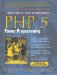# Section 4.3. Iterators

### 4.3. Iterators

The properties of an object can be iterated using the foreach() loop:

` class MyClass {     public \$name = "John";     public \$sex = "male"; } \$obj = new MyClass(); foreach (\$obj as \$key => \$value) {     print "obj[\$key] = \$value\n"; } `

Running this script results in
` obj[name] = John obj[sex] = male `

However, often when you write object-oriented code, your classes don't necessarily represent a simple key/value array as in the previous example, but represent more complex data, such as a database query or a configuration file.

PHP 5 allows you to overload the behavior of the foreach() iteration from within your code so you can have it do what makes sense in respect to your class's design.

Note

Not only does PHP 5 enable you to overload this behavior, but it also allows extension authors to override such behavior, which has brought iterator support to various PHP extensions such as SimpleXML and SQLite.

To overload iteration for your class kind, you need to adhere to certain interfaces that are pre-defined by the language (see Figure 4.2).

##### Figure 4.2. Class diagram of Iterator hierarchy.Any class that implements the traversable interface is a class that can be traversed using the foreach() construct. However, traversable is an empty interface that shouldn't be implemented directly; instead, you should either implement Iterator or IteratorAggregate that inherit from traversable.

The main interface is Iterator. It defines the methods you need to implement to give your classes the foreach() iteration capabilities. These methods should be public and are listed in the following table.

Interface Iterator

void rewind()

Rewinds the iterator to the beginning of the list (this might not always be possible to implement).

mixed current()

Returns the value of the current position.

mixed key()

Returns the key of the current position.

void next()

Moves the iterator to the next key/value pair.

bool valid()

Returns TRue/false if there are more values (used before the call to current() or key()).

If your class implements the Iterator interface, it will be traversable with foreach(). Here's a simple example:

` class NumberSquared implements Iterator {     public function __construct(\$start, \$end)     {         \$this->start = \$start;         \$this->end = \$end;     }     public function rewind()     {         \$this->cur = \$this->start;     }     public function key()     {         return \$this->cur;     }     public function current()     {         return pow(\$this->cur, 2);     }     public function next()     {         \$this->cur++;     }     public function valid()     {         return \$this->cur <= \$this->end;     }     private \$start, \$end;     private \$cur; } \$obj = new NumberSquared(3, 7); foreach (\$obj as \$key => \$value) {     print "The square of \$key is \$value\n"; } `

The output is
` The square of 3 is 9 The square of 4 is 16 The square of 5 is 25 The square of 6 is 36 The square of 7 is 49 `

This example demonstrates how you can implement you own behavior for iterating a class. In this case, the class represents the square of integers, and after given a minimum and maximum value, iterating over those values will give you the number itself and its square.

Now in many cases, your class itself will represent data and have methods to interact with this data. The fact that it also requires an iterator might not be its main functionality. Also, when iterating an object, the state of the iteration (current position) is usually stored in the object itself, thus not allowing for nested iterations. For these two reasons, you may separate the implementation of your class and its iterator by making your class implement the IteratorAggregate interface. Instead of having to define all the previous methods, you need to define a method that returns an object of a different class, which implements the iteration scheme for your class.

The public method you need to implement is Iterator getIterator() because it returns an iterator object that handles the iteration for this class.

By using this method of separating between the class and its iterator, we can rewrite the previous example the following way:

` class NumberSquared implements IteratorAggregate {     public function __construct(\$start, \$end)     {         \$this->start = \$start;         \$this->end = \$end;     }     public function getIterator()     {         return new NumberSquaredIterator(\$this);     }     public function getStart()     {         return \$this->start;     }     public function getEnd()     {         return \$this->end;     }     private \$start, \$end; } class NumberSquaredIterator implements Iterator {     function __construct(\$obj)     {         \$this->obj = \$obj;     }     public function rewind()     {         \$this->cur = \$this->obj->getStart();     }     public function key()     {         return \$this->cur;     }     public function current()     {         return pow(\$this->cur, 2);     }     public function next()     {         \$this->cur++;     }     public function valid()     {         return \$this->cur <= \$this->obj->getEnd();     }     private \$cur;     private \$obj; } \$obj = new NumberSquared(3, 7); foreach (\$obj as \$key => \$value) {     print "The square of \$key is \$value\n"; } `

The output is the same as the previous example. You can clearly see that the IteratorAggregate interface enables you to separate your classes' main functionality and the methods needed for iterating it into two independent entities.

Choose whatever method suits the problem at hand. It really depends on the class and its functionality as to whether the iterator should be in a separate class.PHP 5 Power Programming
ISBN: 013147149X
EAN: 2147483647
Year: 2003
Pages: 240

Similar book on Amazon